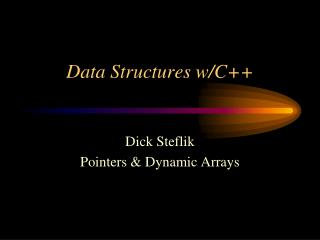Download Presentationteaty

# teaty

Download Presentation## teaty

- - - - - - - - - - - - - - - - - - - - - - - - - - - E N D - - - - - - - - - - - - - - - - - - - - - - - - - - -
##### Presentation Transcript

1. Data Structures w/C++ Dick Steflik Pointers & Dynamic Arrays

2. Static and Dynamic Memory • Static Allocation • allocated by the compiler at compile time • once allocated, does not change • Dynamic Allocation • allocated by program at run time • ‘new’ allocates desired amount from heap • amount depends on class/type • ‘delete’ deallocates an object and returns to storage manager for reallocation

3. Where or When • Static • data stricture sizes are fixed • little chance for storage needs to grow • prototypes • Dynamic • amount of data changes from run to run • data relationships change frequently

4. Pointers • a built-in primitive type; 32 bit • used to hold the storage address of a variable • to define a pointer variable • use the * operator in the definition ex. int *airplane_ptr (airplane_ptr is a variable that will point to an integer)

5. Pointers (cont.) • To assign a value to the pointer variable • use the address operator & ex. int F15; int Airplane_ptr; Airplane_ptr = &F15; Airplane_ptr F15

6. Pointers (cont.) • Note that F15 has not been assigned a value yet • to do this we must use the dereferencing operator * ex. *Airplane_ptr = 5 (dereferencing * reads: location pointed to by var) 5 Airplane_ptr F15

7. Pointers (cont.) • Which is exactly equivalent to: F15 = 5; ...so whats the big deal??

8. The Big Deal.... • We’ve been looking at the trivial case • Pointers to primitives aren’t very useful • things get more interesting with arrays • we can make : • an array that grows as the application needs more room for data • an array that shrinks as the application needs less room for data • and much better with dynamic objects

9. More Pointers int i = 50; int j = 75; int *p1 ; int * p2 p1 = &i ; p2 = & j; cout << *p1; p1 = p2 ; *p2 =0; cout <<*p1;

10. Pointers to arrays • The name of an array is a pointer to the 0th element of the array (the beginning of the array) int array ; // array is equivalent to & array *array = 5; is like array = 5; int j = *(array+4) is like int j = array cout << *array; is like cout << array;